# Mean State

Period Mean (original grids) [Watt m-2]
Model Period Mean (intersection) [Watt m-2]
Model Period Mean (complement) [Watt m-2]
Benchmark Period Mean (intersection) [Watt m-2]
Benchmark Period Mean (complement) [Watt m-2]
Bias [Watt m-2]
RMSE [Watt m-2]
Phase Shift [months]
Bias Score 
RMSE Score 
Seasonal Cycle Score 
Spatial Distribution Score 
Interannual Variability Score 
Overall Score 
Benchmark [-] 179.
CLM4 [-] 191. 191. 177. 216. 13.6 24.0 1.16 0.446 0.450 0.842 0.925 0.514 0.604
CLM4.5 [-] 191. 191. 177. 216. 13.9 24.3 1.14 0.438 0.448 0.847 0.945 0.515 0.607
CLM5 [-] 191. 191. 177. 216. 14.0 24.2 1.11 0.437 0.449 0.853 0.952 0.516 0.609
Period Mean (original grids) [Watt m-2]
Model Period Mean (intersection) [Watt m-2]
Model Period Mean (complement) [Watt m-2]
Benchmark Period Mean (intersection) [Watt m-2]
Benchmark Period Mean (complement) [Watt m-2]
Bias [Watt m-2]
RMSE [Watt m-2]
Phase Shift [months]
Bias Score 
RMSE Score 
Seasonal Cycle Score 
Spatial Distribution Score 
Interannual Variability Score 
Overall Score 
Benchmark [-] 189.
CLM4 [-] 196. 196. 188. 224. 8.04 22.1 1.17 0.591 0.468 0.852 0.890 0.666 0.656
CLM4.5 [-] 197. 197. 188. 224. 8.98 22.6 1.12 0.579 0.463 0.856 0.892 0.678 0.655
CLM5 [-] 198. 198. 188. 224. 9.50 22.8 1.64 0.577 0.456 0.761 0.902 0.671 0.637
Period Mean (original grids) [Watt m-2]
Model Period Mean (intersection) [Watt m-2]
Model Period Mean (complement) [Watt m-2]
Benchmark Period Mean (intersection) [Watt m-2]
Benchmark Period Mean (complement) [Watt m-2]
Bias [Watt m-2]
RMSE [Watt m-2]
Phase Shift [months]
Bias Score 
RMSE Score 
Seasonal Cycle Score 
Spatial Distribution Score 
Interannual Variability Score 
Overall Score 
Benchmark [-] 194.
CLM4 [-] 194. 194. 192. 221. 1.54 22.7 1.16 0.697 0.498 0.842 0.737 0.535 0.634
CLM4.5 [-] 194. 194. 192. 221. 2.04 22.9 1.19 0.691 0.496 0.836 0.729 0.538 0.631
CLM5 [-] 194. 194. 192. 221. 2.03 22.6 1.19 0.694 0.500 0.837 0.751 0.534 0.636
Period Mean (original grids) [Watt m-2]
Model Period Mean (intersection) [Watt m-2]
Model Period Mean (complement) [Watt m-2]
Benchmark Period Mean (intersection) [Watt m-2]
Benchmark Period Mean (complement) [Watt m-2]
Bias [Watt m-2]
RMSE [Watt m-2]
Phase Shift [months]
Bias Score 
RMSE Score 
Seasonal Cycle Score 
Spatial Distribution Score 
Interannual Variability Score 
Overall Score 
Benchmark [-] 81.3
CLM4 [-] 66.2 66.4 80.9 86.2 -15.0 29.1 0.534 0.803 0.678 0.964 0.963 0.690 0.796
CLM4.5 [-] 72.3 72.4 80.9 86.2 -10.0 24.0 0.376 0.852 0.722 0.975 0.836 0.625 0.789
CLM5 [-] 74.9 75.0 80.9 86.2 -7.45 22.7 0.352 0.876 0.732 0.977 0.872 0.607 0.799
Period Mean (original grids) [Watt m-2]
Model Period Mean (intersection) [Watt m-2]
Model Period Mean (complement) [Watt m-2]
Benchmark Period Mean (intersection) [Watt m-2]
Benchmark Period Mean (complement) [Watt m-2]
Bias [Watt m-2]
RMSE [Watt m-2]
Phase Shift [months]
Bias Score 
RMSE Score 
Seasonal Cycle Score 
Spatial Distribution Score 
Interannual Variability Score 
Overall Score 
Benchmark [-] 189.
CLM4 [-] 186. 186. 183. 210. 5.47 26.1 1.54 0.487 0.410 0.772 0.545 0.468 0.515
CLM4.5 [-] 186. 186. 183. 210. 5.47 26.1 1.54 0.487 0.410 0.772 0.543 0.467 0.515
CLM5 [-] 186. 186. 183. 210. 5.12 25.9 1.53 0.491 0.411 0.774 0.550 0.466 0.517
Period Mean (original grids) [Watt m-2]
Model Period Mean (intersection) [Watt m-2]
Model Period Mean (complement) [Watt m-2]
Benchmark Period Mean (intersection) [Watt m-2]
Benchmark Period Mean (complement) [Watt m-2]
Bias [Watt m-2]
RMSE [Watt m-2]
Phase Shift [months]
Bias Score 
RMSE Score 
Seasonal Cycle Score 
Spatial Distribution Score 
Interannual Variability Score 
Overall Score 
Benchmark [-] 105.
CLM4 [-] 108. 108. 104. 127. 3.48 21.7 0.174 0.893 0.754 0.988 0.945 0.621 0.826
CLM4.5 [-] 106. 106. 104. 127. 1.62 23.8 0.179 0.878 0.737 0.988 0.904 0.622 0.811
CLM5 [-] 108. 108. 104. 127. 4.10 20.9 0.174 0.902 0.758 0.988 0.985 0.607 0.833
Period Mean (original grids) [Watt m-2]
Model Period Mean (intersection) [Watt m-2]
Model Period Mean (complement) [Watt m-2]
Benchmark Period Mean (intersection) [Watt m-2]
Benchmark Period Mean (complement) [Watt m-2]
Bias [Watt m-2]
RMSE [Watt m-2]
Phase Shift [months]
Bias Score 
RMSE Score 
Seasonal Cycle Score 
Spatial Distribution Score 
Interannual Variability Score 
Overall Score 
Benchmark [-] 140.
CLM4 [-] 147. 147. 139. 154. 7.90 26.0 0.551 0.742 0.700 0.957 0.964 0.645 0.785
CLM4.5 [-] 147. 146. 139. 154. 7.24 26.1 0.554 0.746 0.696 0.956 0.971 0.640 0.784
CLM5 [-] 149. 148. 139. 154. 8.93 26.1 0.567 0.748 0.694 0.955 0.969 0.641 0.783
Period Mean (original grids) [Watt m-2]
Model Period Mean (intersection) [Watt m-2]
Model Period Mean (complement) [Watt m-2]
Benchmark Period Mean (intersection) [Watt m-2]
Benchmark Period Mean (complement) [Watt m-2]
Bias [Watt m-2]
RMSE [Watt m-2]
Phase Shift [months]
Bias Score 
RMSE Score 
Seasonal Cycle Score 
Spatial Distribution Score 
Interannual Variability Score 
Overall Score 
Benchmark [-] 180.
CLM4 [-] 192. 192. 178. 210. 14.2 31.1 0.423 0.545 0.534 0.970 0.985 0.542 0.685
CLM4.5 [-] 193. 193. 178. 210. 14.8 31.4 0.423 0.538 0.533 0.970 0.978 0.535 0.681
CLM5 [-] 194. 194. 178. 210. 15.4 31.1 0.410 0.539 0.540 0.971 0.961 0.534 0.681
Period Mean (original grids) [Watt m-2]
Model Period Mean (intersection) [Watt m-2]
Model Period Mean (complement) [Watt m-2]
Benchmark Period Mean (intersection) [Watt m-2]
Benchmark Period Mean (complement) [Watt m-2]
Bias [Watt m-2]
RMSE [Watt m-2]
Phase Shift [months]
Bias Score 
RMSE Score 
Seasonal Cycle Score 
Spatial Distribution Score 
Interannual Variability Score 
Overall Score 
Benchmark [-] 74.6
CLM4 [-] 61.4 61.8 74.6 74.8 -13.5 24.7 0.272 0.809 0.689 0.982 0.883 0.684 0.789
CLM4.5 [-] 63.2 63.6 74.6 74.8 -12.1 25.2 0.323 0.818 0.693 0.979 0.781 0.642 0.768
CLM5 [-] 68.2 68.6 74.6 74.8 -7.24 21.8 0.337 0.870 0.717 0.978 0.843 0.618 0.791
Period Mean (original grids) [Watt m-2]
Model Period Mean (intersection) [Watt m-2]
Model Period Mean (complement) [Watt m-2]
Benchmark Period Mean (intersection) [Watt m-2]
Benchmark Period Mean (complement) [Watt m-2]
Bias [Watt m-2]
RMSE [Watt m-2]
Phase Shift [months]
Bias Score 
RMSE Score 
Seasonal Cycle Score 
Spatial Distribution Score 
Interannual Variability Score 
Overall Score 
Benchmark [-] 193.
CLM4 [-] 193. 193. 193. 202. -1.14 20.6 0.475 0.827 0.679 0.967 0.951 0.592 0.782
CLM4.5 [-] 193. 193. 193. 202. -1.07 20.6 0.465 0.826 0.678 0.968 0.943 0.592 0.781
CLM5 [-] 194. 194. 193. 202. -0.170 20.8 0.537 0.822 0.677 0.962 0.954 0.590 0.780
Period Mean (original grids) [Watt m-2]
Model Period Mean (intersection) [Watt m-2]
Model Period Mean (complement) [Watt m-2]
Benchmark Period Mean (intersection) [Watt m-2]
Benchmark Period Mean (complement) [Watt m-2]
Bias [Watt m-2]
RMSE [Watt m-2]
Phase Shift [months]
Bias Score 
RMSE Score 
Seasonal Cycle Score 
Spatial Distribution Score 
Interannual Variability Score 
Overall Score 
Benchmark [-] 120.
CLM4 [-] 130. 130. 117. 151. 13.5 28.5 0.275 0.740 0.740 0.982 0.981 0.663 0.808
CLM4.5 [-] 131. 131. 117. 151. 14.4 28.0 0.268 0.743 0.741 0.982 0.994 0.655 0.809
CLM5 [-] 131. 131. 117. 151. 14.4 27.6 0.295 0.744 0.747 0.980 0.995 0.642 0.809
Period Mean (original grids) [Watt m-2]
Model Period Mean (intersection) [Watt m-2]
Model Period Mean (complement) [Watt m-2]
Benchmark Period Mean (intersection) [Watt m-2]
Benchmark Period Mean (complement) [Watt m-2]
Bias [Watt m-2]
RMSE [Watt m-2]
Phase Shift [months]
Bias Score 
RMSE Score 
Seasonal Cycle Score 
Spatial Distribution Score 
Interannual Variability Score 
Overall Score 
Benchmark [-] 168.
CLM4 [-] 141. 141. 143. 180. -1.23 24.4 0.585 0.705 0.629 0.936 0.953 0.563 0.736
CLM4.5 [-] 142. 142. 143. 180. -0.716 24.0 0.590 0.710 0.631 0.935 0.959 0.557 0.737
CLM5 [-] 143. 143. 143. 180. -0.0159 23.9 0.610 0.714 0.632 0.932 0.956 0.554 0.736
Period Mean (original grids) [Watt m-2]
Model Period Mean (intersection) [Watt m-2]
Model Period Mean (complement) [Watt m-2]
Benchmark Period Mean (intersection) [Watt m-2]
Benchmark Period Mean (complement) [Watt m-2]
Bias [Watt m-2]
RMSE [Watt m-2]
Phase Shift [months]
Bias Score 
RMSE Score 
Seasonal Cycle Score 
Spatial Distribution Score 
Interannual Variability Score 
Overall Score 
Benchmark [-] 175.
CLM4 [-] 166. 166. 173. 224. -6.43 21.8 0.376 0.702 0.698 0.969 0.996 0.580 0.774
CLM4.5 [-] 167. 167. 173. 224. -5.73 21.6 0.378 0.704 0.699 0.969 0.996 0.581 0.774
CLM5 [-] 168. 168. 173. 224. -4.48 21.6 0.402 0.705 0.696 0.965 0.996 0.590 0.775
Period Mean (original grids) [Watt m-2]
Model Period Mean (intersection) [Watt m-2]
Model Period Mean (complement) [Watt m-2]
Benchmark Period Mean (intersection) [Watt m-2]
Benchmark Period Mean (complement) [Watt m-2]
Bias [Watt m-2]
RMSE [Watt m-2]
Phase Shift [months]
Bias Score 
RMSE Score 
Seasonal Cycle Score 
Spatial Distribution Score 
Interannual Variability Score 
Overall Score 
Benchmark [-] 80.0
CLM4 [-] 72.6 72.4 79.8 82.9 -9.25 23.2 0.161 0.834 0.725 0.989 0.789 0.673 0.789
CLM4.5 [-] 74.2 74.0 79.8 82.9 -7.98 22.6 0.159 0.848 0.728 0.989 0.768 0.631 0.782
CLM5 [-] 75.4 75.2 79.8 82.9 -6.91 22.0 0.127 0.855 0.732 0.992 0.792 0.624 0.788
Period Mean (original grids) [Watt m-2]
Model Period Mean (intersection) [Watt m-2]
Model Period Mean (complement) [Watt m-2]
Benchmark Period Mean (intersection) [Watt m-2]
Benchmark Period Mean (complement) [Watt m-2]
Bias [Watt m-2]
RMSE [Watt m-2]
Phase Shift [months]
Bias Score 
RMSE Score 
Seasonal Cycle Score 
Spatial Distribution Score 
Interannual Variability Score 
Overall Score 
Benchmark [-] 205.
CLM4 [-] 189. 189. 200. 227. -8.80 29.1 0.946 0.601 0.585 0.895 0.719 0.529 0.652
CLM4.5 [-] 189. 189. 200. 227. -9.47 28.9 0.917 0.607 0.585 0.898 0.655 0.530 0.643
CLM5 [-] 189. 189. 200. 227. -9.36 28.6 0.810 0.611 0.588 0.919 0.631 0.527 0.644
Period Mean (original grids) [Watt m-2]
Model Period Mean (intersection) [Watt m-2]
Model Period Mean (complement) [Watt m-2]
Benchmark Period Mean (intersection) [Watt m-2]
Benchmark Period Mean (complement) [Watt m-2]
Bias [Watt m-2]
RMSE [Watt m-2]
Phase Shift [months]
Bias Score 
RMSE Score 
Seasonal Cycle Score 
Spatial Distribution Score 
Interannual Variability Score 
Overall Score 
Benchmark [-] 153.
CLM4 [-] 157. 157. 151. 194. 7.68 20.0 0.458 0.826 0.747 0.969 0.998 0.544 0.805
CLM4.5 [-] 156. 157. 151. 194. 6.80 19.7 0.456 0.844 0.743 0.970 0.999 0.553 0.809
CLM5 [-] 156. 156. 151. 194. 6.08 19.4 0.518 0.854 0.744 0.966 0.999 0.546 0.809
Period Mean (original grids) [Watt m-2]
Model Period Mean (intersection) [Watt m-2]
Model Period Mean (complement) [Watt m-2]
Benchmark Period Mean (intersection) [Watt m-2]
Benchmark Period Mean (complement) [Watt m-2]
Bias [Watt m-2]
RMSE [Watt m-2]
Phase Shift [months]
Bias Score 
RMSE Score 
Seasonal Cycle Score 
Spatial Distribution Score 
Interannual Variability Score 
Overall Score 
Benchmark [-] 115.
CLM4 [-] 122. 122. 114. 123. 7.44 20.7 0.241 0.861 0.783 0.984 0.913 0.588 0.819
CLM4.5 [-] 123. 123. 114. 123. 8.38 20.3 0.248 0.863 0.786 0.984 0.951 0.566 0.822
CLM5 [-] 123. 123. 114. 123. 8.13 20.1 0.223 0.869 0.784 0.985 0.975 0.573 0.828
Period Mean (original grids) [Watt m-2]
Model Period Mean (intersection) [Watt m-2]
Model Period Mean (complement) [Watt m-2]
Benchmark Period Mean (intersection) [Watt m-2]
Benchmark Period Mean (complement) [Watt m-2]
Bias [Watt m-2]
RMSE [Watt m-2]
Phase Shift [months]
Bias Score 
RMSE Score 
Seasonal Cycle Score 
Spatial Distribution Score 
Interannual Variability Score 
Overall Score 
Benchmark [-] 162.
CLM4 [-] 153. 154. 162. 171. -7.84 19.3 0.270 0.877 0.782 0.981 0.989 0.631 0.840
CLM4.5 [-] 152. 153. 162. 171. -9.02 19.6 0.275 0.864 0.785 0.981 0.995 0.622 0.839
CLM5 [-] 155. 155. 162. 171. -6.38 18.0 0.279 0.895 0.790 0.981 0.991 0.615 0.844
Period Mean (original grids) [Watt m-2]
Model Period Mean (intersection) [Watt m-2]
Model Period Mean (complement) [Watt m-2]
Benchmark Period Mean (intersection) [Watt m-2]
Benchmark Period Mean (complement) [Watt m-2]
Bias [Watt m-2]
RMSE [Watt m-2]
Phase Shift [months]
Bias Score 
RMSE Score 
Seasonal Cycle Score 
Spatial Distribution Score 
Interannual Variability Score 
Overall Score 
Benchmark [-] 176.
CLM4 [-] 182. 182. 174. 226. 8.36 22.4 1.45 0.642 0.431 0.789 0.810 0.478 0.597
CLM4.5 [-] 182. 182. 174. 226. 8.41 22.5 1.46 0.639 0.430 0.787 0.796 0.478 0.594
CLM5 [-] 182. 182. 174. 226. 8.23 22.4 1.44 0.644 0.432 0.791 0.788 0.477 0.594
Period Mean (original grids) [Watt m-2]
Model Period Mean (intersection) [Watt m-2]
Model Period Mean (complement) [Watt m-2]
Benchmark Period Mean (intersection) [Watt m-2]
Benchmark Period Mean (complement) [Watt m-2]
Bias [Watt m-2]
RMSE [Watt m-2]
Phase Shift [months]
Bias Score 
RMSE Score 
Seasonal Cycle Score 
Spatial Distribution Score 
Interannual Variability Score 
Overall Score 
Benchmark [-] 182.
CLM4 [-] 185. 185. 181. 201. 3.56 23.8 1.05 0.776 0.525 0.867 0.906 0.485 0.681
CLM4.5 [-] 185. 185. 181. 201. 3.54 23.8 1.14 0.775 0.525 0.847 0.912 0.487 0.679
CLM5 [-] 184. 184. 181. 201. 2.76 23.3 0.997 0.780 0.530 0.872 0.927 0.480 0.686
Period Mean (original grids) [Watt m-2]
Model Period Mean (intersection) [Watt m-2]
Model Period Mean (complement) [Watt m-2]
Benchmark Period Mean (intersection) [Watt m-2]
Benchmark Period Mean (complement) [Watt m-2]
Bias [Watt m-2]
RMSE [Watt m-2]
Phase Shift [months]
Bias Score 
RMSE Score 
Seasonal Cycle Score 
Spatial Distribution Score 
Interannual Variability Score 
Overall Score 
Benchmark [-] 176.
CLM4 [-] 174. 174. 176. 178. -3.97 28.3 0.521 0.715 0.666 0.950 0.829 0.514 0.723
CLM4.5 [-] 175. 174. 176. 178. -3.47 28.4 0.517 0.715 0.664 0.950 0.820 0.517 0.722
CLM5 [-] 176. 175. 176. 178. -2.14 28.3 0.529 0.714 0.664 0.949 0.810 0.511 0.719

# Temporally integrated period mean

BENCHMARK MEAN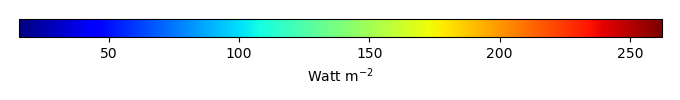MODEL MEANBIAS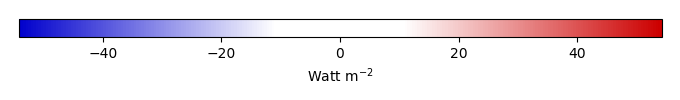BIAS SCORERMSE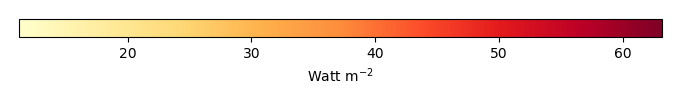RMSE SCOREBENCHMARK INTERANNUAL VARIABILITY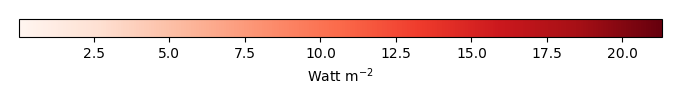MODEL INTERANNUAL VARIABILITYINTERANNUAL VARIABILITY SCOREBENCHMARK MAX MONTHMODEL MAX MONTHDIFFERENCE IN MAX MONTH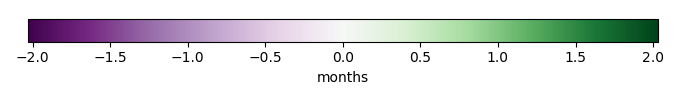SEASONAL CYCLE SCORESPATIAL TAYLOR DIAGRAMMODEL COLORS# Spatially integrated regional mean

MODEL COLORSREGIONAL MEANANNUAL CYCLEMONTHLY ANOMALYANNUAL CYCLE# All Models

BenchmarkCLM4CLM4.5CLM5# Data Information

creation_date: Tue Jul 1 08:29:23 PDT 2014

source_file: This product is generated from monthly 1 degree GEWEX SRB Radiation observations